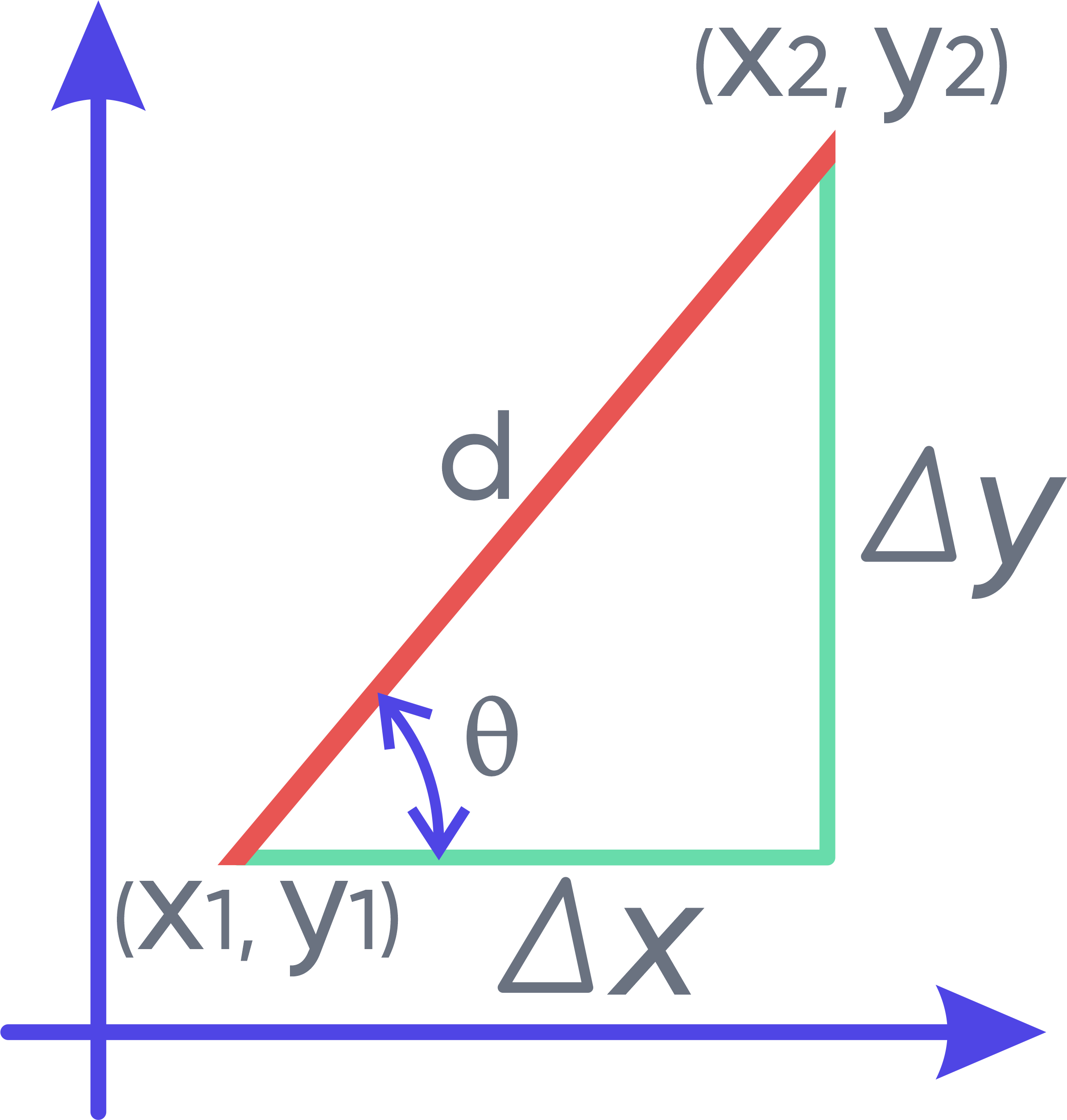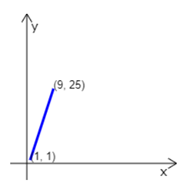Math Calculators
Slope Calculator

# Slope Calculator

The slope calculator finds the slope of a line using the slope formula. It can also find point coordinates, incline angle, and length if the slope and one point are known.

Slope
Slope (m) 1.75
Distance (d) 8.062258
Delta x (Δx) 4
Delta y (Δy) 7

There was an error with your calculation.## Slope calculator

The slope calculator is a straightforward online tool allowing you to find the slope of a straight line. In mathematics, the slope of a line is defined as the change in the vertical coordinate (y-coordinate) relative to the change in the horizontal coordinate (x-coordinate).

## Used notationThe slope is denoted by the letter m. The plot above graphically demonstrates all other notations used in the calculator. The slope finder can perform calculations in two different scenarios:

1. When the coordinates of the two points on the line are known. On the graph, the two points have the coordinates (x₁,y₁) and (x₂,y₂). In this case, the calculator will find the slope of the line, m.

2. If we know the coordinates of one point (x₁,y₁), the distance d and the slope of a line, the calculator will find the coordinates of the second point on the line, (x₂,y₂).

In both scenarios, the calculator will also return other missing characteristics of the line: the horizontal change ∆x, the vertical change ∆y, the inclination angle θ, the line length, or distance, d.

## Directions for use

First, identify the known values and choose the appropriate calculator. If the coordinates of the two points are known, select “If the 2 Points are known.”

If you have only the coordinates of one of the points, to perform calculations, you will need to know the distance, d, and the slope of the line, m. In this case, choose “If 1 Point and the Slope are known.”

## If the 2 Points are Known

Insert the known coordinates of the points in the corresponding fields, then press “Calculate.” The calculator will return the following information:

• the slope m,
• the inclination angle θ,
• the length of the line d,
• the horizontal change ∆x,
• the vertical change ∆y.

The calculator will also demonstrate the formulas used to find the slope and all other characteristic values of the line. The calculator will display the corresponding equation of the line, and it will schematically plot the line for visual representation.

## If 1 Point and the Slope are Known

Insert the known coordinates of the point, the distance, and the slope to the corresponding fields. Note that instead of the slope, you can insert the value of the “angle of incline (theta or θ).” The value of θ has to be inserted in degrees. Only one of these values has to be inserted (either m or θ). Suppose both m and θ are inserted. In that case, the calculator will ignore the value of θ, and only use the slope m for the calculations.

Press “Calculate.” The calculator will return the following information: the coordinates of the second point (x₂,y₂), the horizontal change ∆x, the vertical change ∆y, and the length of the line d. If the slope m was used for the calculations, the calculator would also return the value of θ. If you used the angle of incline θ for the calculations, the calculator would return the value of m in the answer. Also, the calculator will display the corresponding equation of the line, and it will schematically plot the line for visual representation.

## Slope formula

As mentioned above, the slope of a line is defined as the change in the vertical coordinate (y-coordinate) of a line relative to the change in the horizontal coordinate (x-coordinate):

$$m=\frac{y₂-y₁}{x₂-x₁}=\frac{∆y}{∆x}=tanθ$$

The equation above is called the slope formula. We can use it to find the slope of any given line if the coordinates of two points on the line are known. The slope is commonly denoted as m. It is used to describe the direction of the line, as well as its steepness:

• If the line goes upwards from left to right, then y₂>y₁ when x₂>x₁. The slope will always be positive, m>0. In this case, we say that the line is increasing.

• If the line goes downwards from left to right, then then y₂ < y₁ when x₂ > x₁. The slope will be negative, m < 0. In this case, we say that the line is decreasing.

• If the line is horizontal, then y₂=y₁ and y₂-y₁=0. Then the slope will also equal zero: m=0.

• If the line is vertical, then x₂=x₁ and x₂-x₁=0. The slope formula will have a zero in the denominator, and the slope is undefined.

## Line equation

We can write any linear equation in the following form:

$$y=mx+b$$

This form of linear equation is called the slope-intercept form. The plot of this equation will be a straight line, where m is the slope of the line. And B is the coordinate at which the graph intercepts the y-axis. B is sometimes also called the y-intercept of the line, since y=b when x=0.

When the coordinates of one point on the line and the slope are known, we can write the line equation in the so-called point-slope form:

$$y-y₁=m(x-x₁)$$

This form of the linear equation is beneficial for finding the y-intercept of a line.

## Calculation example

Let’s assume we know the coordinates of the two points on the line.

Given:

$$x₁=1$$

$$y₁=1$$

$$x₂=9$$

$$y₂=25$$

Let’s first find the slope of this line:

$$m=\frac{y₂-y₁}{x₂-x₁}=\frac{∆y}{∆x}$$

$$m=\frac{25-1}{9-1}=\frac{24}{8}=3$$

$$m=3$$

Now, let’s find the other characteristic values of the line. We know that m=tanθ. Therefore, we can find the angle of incline θ as follows:

$$\theta=\arctan{\left(m\right)} = arctan\frac{∆x}{∆y} = 71.565051177078°$$

Furthermore,

$$∆x=9-1=8$$

$$∆y=25-1=24$$

We can find the distance d using a Pythagorean theorem. It states that the square of the length of the hypotenuse equals the sum of the squares of the length of the legs of the right triangle.Applying this theorem to our triangle, we get:

$$d^2=∆x2+∆y2$$

Therefore,

$$d=∆x2+∆y2$$

$$d=\sqrt{8^2+{24}^2}=\sqrt{640}$$

$$d=25.298221281347$$

To find the y-intercept of the line, let’s write the line equation in the point-slope form, substituting our given values of m, x₁, and y₁:

$$y-1=3\left(x-1\right)$$

$$y=3x-2$$

Therefore, y=-2 is the y-intercept of the line, or, in other words, when x=0, y=-2.

If y=0:

$$x=\frac{2}{3}=0.66666666666667$$The sketch demonstrates the corresponding line. In our case, the slope is positive, m>0, and we can see that the line is increasing – it goes up from left to right. We can also see that the line is rather steep since the inclination angle θ ≈ 72°.